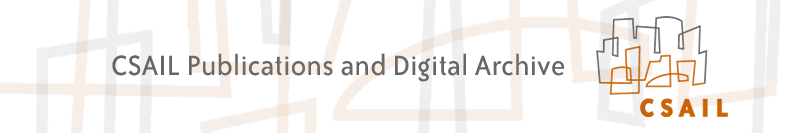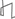Research Abstracts Home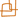CSAIL Digital Archive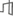Research Activities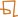CSAIL HomeResearch Abstracts - 2007IntroductionPerception & LearningPhysical, Biological & Social SystemsSystemsTheory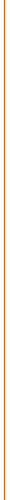### Limits to List Decoding Reed-Solomon Codes

#### Eli Ben-Sasson, Swastik Kopparty & Jaikumar Radhakrishnan

A fundamental task associated with the use of error-correcting codes is {\em decoding,} that is, recovering the original codeword $w$, from the corrupted received word $w'$. In {\em list decoding}, introduced independently by Elias and Wozencraft, we consider a relaxation of the above task. We no longer demand that the codeword $w$ be recovered uniquely. Instead, we ask for a of possible codewords, and we call the recovery successful if $w$ appears in this list. The crucial point is that list decoding (with relatively small lists) is possible even when the received word's agreement with the original word is so small that any hope of recovering the original word uniquely is unrealistic.

Clearly, the size of the list is a crucial parameter of the problem (to wit, the set of all codewords is a trivial solution for unbounded list size). In order to quantify the number of errors one can tolerate for list decoding and appreciate the difference with unique decoding, let us consider a code with block length $N$ and distance $d$. Suppose the number of errors in the transmission is guaranteed to be at most $e$. Then, in the worst case, we can uniquely recover the original message only if $e$ is at most $\frac{d-1}{2}$. Thus, even if $d$ is very close to $N$, we require that the two words agree on at least $N - \frac{d-1}{2} \geq \frac{N}{2}$ places. With list decoding we can, in principle, tolerate substantially more errors.

\begin{theorem}[Johnson bound \cite{Johnson,Johnson1,Guruswami-Sudan-JB}] Let ${\cal C}$ be a code with block length $N$ and distance $d$. Then, the number of codewords that agree with any word $w'$ on more than $\sqrt{N(N-d)}$ places is $O(N^2)$. \end{theorem}

For example, if the code has distance $0.99N$, then there are only a polynomial number of words that agree with the given word on more than $\frac{N}{10}$ places, that is, we have polynomial size lists as long as the error is less than $0.9N$. In fact, a more precise form of the Johnson bound~\cite{Guruswami-Sudan-JB} implies that if the error is less than $0.8N$, then there are only a constant number of such codewords. In contrast, unique decoding is feasible only when the error is guaranteed to be less than $0.495N$. Given a specific family of codes (in this paper, we shall consider Reed-Solomon codes), we recall two fundamental problems associated with list decoding:

Combinatorial list decoding:
Given code ${\cal{C}}$ of block-length $N$ and agreement parameter $A\leq N$, estimate the maximal number of distinct codewords that agree with $w'$ on at least $A$ entries, where the maximum is taken over all (possibly corrupted) received words $w'$.
Algorithmic list decoding:
Devise an efficient algorithm for ${\cal {C}}$ that on input $w'$ and agreement parameter $A$, lists all words that agree with $w'$ on at least $A$ entries.

In order to solve the algorithmic list decoding problem (for a specific code ${\cal{C}}$ and agreement parameter $A$) in worst-case time $T$, at the very least we should have a combinatorial guarantee that there are no more than $T$ codewords in the list. In other words, lower bounds for the combinatorial problem imply lower bounds for the algorithmic one.

Our main result is an improved lower bound for the combinatorial list decoding problem for Reed-Solomon codes (described next). This result implies super-polynomial lower bounds on the running time of any list decoding algorithm for Reed-Solomon codes for a sufficiently small agreement parameter.

##### References:

 Eli Ben-Sasson, Swastik Kopparty and Jaikumar Radhakrishnan. Subspace polynomials and List Decoding of Reed-Solomon Codes. In Proceedings of FOCS 2006, Berkeley, CA, 2006.Computer Science and Artificial Intelligence Laboratory (CSAIL) The Stata Center, Building 32 - 32 Vassar Street - Cambridge, MA 02139 - USA tel:+1-617-253-0073 - publications@csail.mit.edu